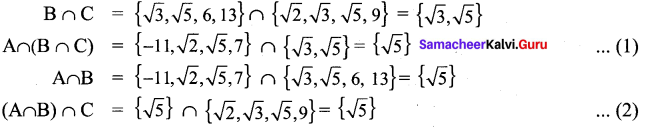## Tamilnadu Samacheer Kalvi 9th Maths Solutions Chapter 1 Set Language Ex 1.4

Exercise 1.4 Class 9 Maths Samacheer Question 1.
If P= {1, 2, 5, 7, 9}, Q = {2, 3, 5, 9, 11}, R = {3, 4, 5, 7, 9} and S = {2, 3, 4, 5, 8}, then find
(i) (P ∪ Q) ∪ R
(ii) (P ∩ Q) ∩ S
(iii) (Q ∩ S) ∩ R
Solution:
(i) (P ∪ Q) ∪ R
(P ∪ Q) = {1, 2, 5, 7, 9} ∪ {2, 3, 5, 9, 11} = {1, 2, 3, 5, 7, 9, 11}
(P ∪ Q) ∪ R = {1, 2, 3, 5, 7, 9, 11} ∪ {3, 4, 5, 7, 9} = {1, 2, 3, 4, 5, 7, 9, 11}

(ii)(P ∩ Q) ∩ S
(P ∩ Q) = {1, 2, 5, 7, 9} ∩ {2, 3, 5, 9, 11} = {2, 5, 9}
(P ∩ Q) ∩ S = {2, 5, 9} ∩ {2, 3, 4, 5, 8} = {2, 5}

(iii) (Q ∩ S) ∩ R
(Q ∩ S) = {2, 3, 5, 9, 11} ∩ {2, 3, 4, 5, 8} = {2, 3, 5}
(Q ∩ S) ∩ R = {2, 3, 5} ∩ {3, 4, 5, 7, 9} = {3, 5}

Exercise 1.4 Class 9 Maths Solution Samacheer Question 2.
Test for the commutative property of union and intersection of the sets
P = {x : x is a real number between 2 and 7} and
Q = {x : x is an irrational number between 2 and 7}
Solution:
Commutative Property of union of sets
(A ∪ B)’ = (B ∪ A)
Here P = {3, 4, 5, 6}, Q = {$$\sqrt{3}, \sqrt{5}, \sqrt{6}$$}
P ∪ Q = {3, 4, 5, 6} ∪ {$$\sqrt{3}, \sqrt{5}, \sqrt{6}$$} = {3, 4, 5, 6, $$\sqrt{3}, \sqrt{5}, \sqrt{6}$$} …………. (1)
Q ∪ P = {$$\sqrt{3}, \sqrt{5}, \sqrt{6}$$} ∪ {3, 4, 5, 6}= {$$\sqrt{3}, \sqrt{5}, \sqrt{6}$$, 3, 4, 5, 6} ………… (2)
(1) = (2)
∴ P ∪ Q = Q ∪ P
∴ It is verified that union of sets is commutative.
Commutative Property of intersection of sets (P ∩ Q) = (Q ∩ P)
P ∩ Q = {3, 4, 5, 6} ∩ {$$\sqrt{3}, \sqrt{5}, \sqrt{6}$$} = { } ………. (1)
Q ∩ P = {$$\sqrt{3}, \sqrt{5}, \sqrt{6}$$} ∩ {3, 4, 5, 6} = { } …………. (2)
From (1) and (2)
P ∩ Q = Q ∩ P
∴ It is verified that intersection of sets is commutative.

Samacheer Kalvi 9th Maths Exercise 1.4 Question 3.
If A = {p, q, r, s}, B = {m, n, q, s, t} and C = {m, n, p, q, s}, then verify the associative property of union of sets.
Solution:
Associative Property of union of sets
A ∪ (B ∪ C) = (A ∪ B) ∪ C)
B ∪ C = {m, n, q, s, t} ∪ {m, n,p, q, s}= {m, n, p, q, s, t}
A ∪ (B ∪ C) = {p, q, r, s} ∪ {m, n, p, q, s, t} = {m, n, p, q, r, s, t} ………..… (1)
(A ∪ B) = {p, q, r, s} ∪ {m, n, q, s, t} = {p, q, r, s, m, n, t}
(A ∪ B) ∪ C = {p, q, r, s, m, n, t} ∪ {m, n, p, q, s} = {p, q, r, s, m, n, t} …………… (2)
From (1) & (2)
It is verified that A ∪ (B ∪ C) = (A ∪ B) ∪ C

9th Standard Maths Exercise 1.4 Question 4.
Verify the associative property of intersection of sets for A = {-11, $$\sqrt{2}, \sqrt{5}$$ ,7}, B = {$$\sqrt{3}, \sqrt{5}$$, 6, 13} and C = {$$\sqrt{2}, \sqrt{3}, \sqrt{5}$$, 9}.
Solution:
Associative Property of intersection of sets A ∩ (B ∩ C) = (A ∩ B) ∩ C)From (1) and (2), it is verified that A ∩ (B ∩ C) = (A ∩ B) ∩ C

9th Maths Exercise 1.4 Question 5.
If A = {x : x = 2n, n ∈ W and n < 4}, B = {x: x = 2 n, n ∈ N and n ≤ 4} and C = {0, 1, 2, 5, 6}, then verify the associative property of intersection of sets.
Solution:
A = {x : x = 2n, n ∈ W, n < 4}
⇒ x = 2°= 1
x = 21 = 2
x = 22 = 4
x = 23 = 8
∴ A = {1, 2, 4, 8}

B = {x : x = 2n, n ∈ N and n ≤ 4}
⇒ x = 2 × 1 = 2
x = 2 × 2 = 4
x = 2 × 3 = 6
x = 2 × 4 = 8
∴ B = {2, 4, 6, 8}
C = {0, 1, 2, 5, 6}
Associative property of intersection of sets
A ∩ (B ∩ C) = (A ∩ B) ∩ C
B ∩ C = {2, 6}
A ∩ (B ∩ C) = {1, 2, 4, 8} ∩ {2, 6} = {2} ………..… (1)
A ∩ B = {1, 2, 4, 8} ∩ {2, 4, 6, 8} = {2, 4, 8}
(A ∩ B) ∩ C = {2, 4, 8} ∩ {0, 1, 2, 5, 6} = {2} …………….. (2)
From (1) and (2), It is verified that A ∩ (B ∩ C) = (A ∩ B) ∩ C